Case Based Questions Test: Triangles - 2

# Case Based Questions Test: Triangles - 2 - Class 10

Test Description

## 10 Questions MCQ Test Mathematics (Maths) Class 10 - Case Based Questions Test: Triangles - 2

Case Based Questions Test: Triangles - 2 for Class 10 2023 is part of Mathematics (Maths) Class 10 preparation. The Case Based Questions Test: Triangles - 2 questions and answers have been prepared according to the Class 10 exam syllabus.The Case Based Questions Test: Triangles - 2 MCQs are made for Class 10 2023 Exam. Find important definitions, questions, notes, meanings, examples, exercises, MCQs and online tests for Case Based Questions Test: Triangles - 2 below.
Solutions of Case Based Questions Test: Triangles - 2 questions in English are available as part of our Mathematics (Maths) Class 10 for Class 10 & Case Based Questions Test: Triangles - 2 solutions in Hindi for Mathematics (Maths) Class 10 course. Download more important topics, notes, lectures and mock test series for Class 10 Exam by signing up for free. Attempt Case Based Questions Test: Triangles - 2 | 10 questions in 20 minutes | Mock test for Class 10 preparation | Free important questions MCQ to study Mathematics (Maths) Class 10 for Class 10 Exam | Download free PDF with solutions
 1 Crore+ students have signed up on EduRev. Have you?
Case Based Questions Test: Triangles - 2 - Question 1

### Direction: Read the following text and answer the below questions:SIMILAR TRIANGLESVijay is trying to find the average height of a tower near his house. He is using the properties of similar triangles. The height of Vijay’s house if 20 m when Vijay’s house casts a shadow 10 m long on the ground. At the same time, the tower casts a shadow 50 m long on the ground and the house of Ajay casts 20 m shadow on the ground.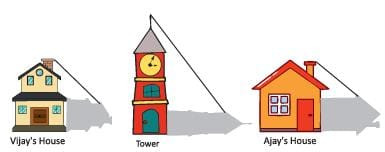When the tower casts a shadow of 40 m, same time what will be the length of the shadow of Vijay’s house?

Detailed Solution for Case Based Questions Test: Triangles - 2 - Question 1
The height of Vijay’s house is AC = 20m.

The length of shadow of the tower is A’B’= 40m.

The height of tower is A’C’= 100m.

The length of the shadow of Vijay ′ s house is AB.

∵ ΔABC & ΔA′B′C ′ are similar triangles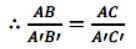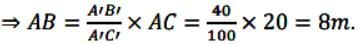∴ The length of the shadow of Vijay ′ s house is 8m.

Case Based Questions Test: Triangles - 2 - Question 2

### Direction: Read the following text and answer the below questions:SIMILAR TRIANGLESVijay is trying to find the average height of a tower near his house. He is using the properties of similar triangles. The height of Vijay’s house if 20 m when Vijay’s house casts a shadow 10 m long on the ground. At the same time, the tower casts a shadow 50 m long on the ground and the house of Ajay casts 20 m shadow on the ground.What will be the length of the shadow of the tower when Vijay’s house casts a shadow of 12 m?

Detailed Solution for Case Based Questions Test: Triangles - 2 - Question 2
The height of Vijay’s house is AC = 20m.

The height of the tower is A’C’ =100m.

The length of shadow of Vijay’s house is AB = 12cm.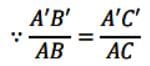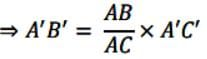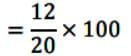= 12 x 5

= 60 m

Case Based Questions Test: Triangles - 2 - Question 3

### Direction: Read the following text and answer the below questions:SIMILAR TRIANGLESVijay is trying to find the average height of a tower near his house. He is using the properties of similar triangles. The height of Vijay’s house if 20 m when Vijay’s house casts a shadow 10 m long on the ground. At the same time, the tower casts a shadow 50 m long on the ground and the house of Ajay casts 20 m shadow on the ground.When the tower casts a shadow of 40 m, same time what will be the length of the shadow of Ajay’s house?

Detailed Solution for Case Based Questions Test: Triangles - 2 - Question 3
The height of tower is A’C’= 100m.

And the height of Ajay’s house is PR = 40m

The length of shadow of tower is A’B’= 40m.

The length of shadow of Ajay’s house is PQ.

∵ ΔA′B′C′ and ΔPQR are similar triangles.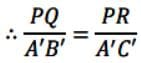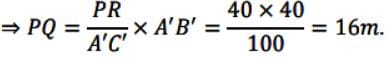Hence, the length of shadow of Ajay’s house is 16m.

Case Based Questions Test: Triangles - 2 - Question 4

SIMILAR TRIANGLES

Vijay is trying to find the average height of a tower near his house. He is using the properties of similar triangles. The height of Vijay’s house if 20 m when Vijay’s house casts a shadow 10 m long on the ground. At the same time, the tower casts a shadow 50 m long on the ground and the house of Ajay casts 20 m shadow on the ground.What is the height of the tower?

Detailed Solution for Case Based Questions Test: Triangles - 2 - Question 4
When two corresponding angles of two triangles are similar, then ratio of sides are equal.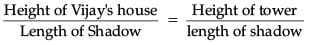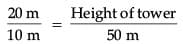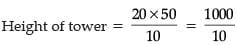= 100 m.

Case Based Questions Test: Triangles - 2 - Question 5

SIMILAR TRIANGLES

Vijay is trying to find the average height of a tower near his house. He is using the properties of similar triangles. The height of Vijay’s house if 20 m when Vijay’s house casts a shadow 10 m long on the ground. At the same time, the tower casts a shadow 50 m long on the ground and the house of Ajay casts 20 m shadow on the ground.What is the height of Ajay’s house?

Detailed Solution for Case Based Questions Test: Triangles - 2 - Question 5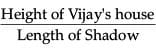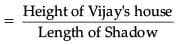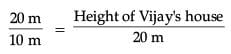Height of Ajay's house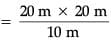= 40 m.

Case Based Questions Test: Triangles - 2 - Question 6

Direction: Read the following text and answer the following questions on the basis of the same:

SCALE FACTOR AND SIMILARITY SCALE FACTOR

A scale drawing of an object is of the same shape as the object but of a different size.

The scale of a drawing is a comparison of the length used on a drawing to the length it represents. The value of scale is written as a ratio.

SIMILAR FIGURES

The ratio of two corresponding sides in similar figures is called the scale factor.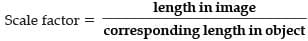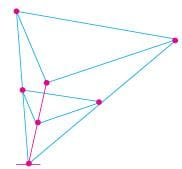If one shape can become another using Resizing then the shapes are Similar.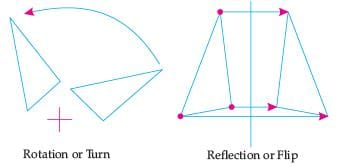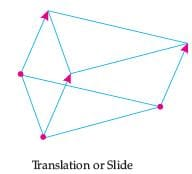Hence, two shapes are Similar when one can become the other after a resize, flip, slide or turn.

Below you see a student's mathematical model of a farmhouse roof with measurements. The attic floor, ABCD in the model, is a square. The beams that support the roof are the edge of a rectangular prism, EFGHKLMN.

E is the middle of AT, F is the middle of BT, G is the middle of CT, and H is the middle of DT. All the edges of the pyramid in the model have length of 12 m.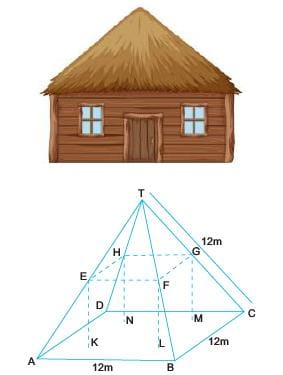What is the length of EF, where EF is one of the horizontal edges of the block ?

Detailed Solution for Case Based Questions Test: Triangles - 2 - Question 6
Length of the horizontal edge EF

= half of the edge of pyramid

= 12/2

= 6 cm (as E is the mid-point of AT)

Case Based Questions Test: Triangles - 2 - Question 7

Direction: Read the following text and answer the following questions on the basis of the same:

SCALE FACTOR AND SIMILARITY SCALE FACTOR

A scale drawing of an object is of the same shape as the object but of a different size.

The scale of a drawing is a comparison of the length used on a drawing to the length it represents. The value of scale is written as a ratio.

SIMILAR FIGURES

The ratio of two corresponding sides in similar figures is called the scale factor.If one shape can become another using Resizing then the shapes are Similar.Hence, two shapes are Similar when one can become the other after a resize, flip, slide or turn.

What will effect the similarity of any two polygons ?

Detailed Solution for Case Based Questions Test: Triangles - 2 - Question 7
They are not the mirror image of one another.
Case Based Questions Test: Triangles - 2 - Question 8

Direction: Read the following text and answer the following questions on the basis of the same:
SCALE FACTOR AND SIMILARITY SCALE FACTOR
A scale drawing of an object is of the same shape as the object but of a different size.
The scale of a drawing is a comparison of the length used on a drawing to the length it represents. The value of scale is written as a ratio.
SIMILAR FIGURES
The ratio of two corresponding sides in similar figures is called the scale factor.If one shape can become another using Resizing then the shapes are Similar.Hence, two shapes are Similar when one can become the other after a resize, flip, slide or turn.

The shadow of a stick 5 m long is 2 m. At the same time the shadow of a tree 12.5 m high is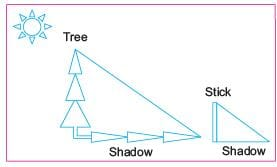Detailed Solution for Case Based Questions Test: Triangles - 2 - Question 8

Let shadow of the tree be x.

By the property to similar triangles we have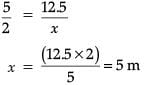Case Based Questions Test: Triangles - 2 - Question 9

Direction: Read the following text and answer the following questions on the basis of the same:

SCALE FACTOR AND SIMILARITY SCALE FACTOR

A scale drawing of an object is of the same shape as the object but of a different size.

The scale of a drawing is a comparison of the length used on a drawing to the length it represents. The value of scale is written as a ratio.

SIMILAR FIGURES

The ratio of two corresponding sides in similar figures is called the scale factor.If one shape can become another using Resizing then the shapes are Similar.Hence, two shapes are Similar when one can become the other after a resize, flip, slide or turn.

A model of a boat is made on the scale of 1 : 4. The model is 120 cm long. The full size of the boat has a width of 60 cm. What is the width of the scale model ?Detailed Solution for Case Based Questions Test: Triangles - 2 - Question 9
Width of the scale model = 60/4

= 15 cm.

Case Based Questions Test: Triangles - 2 - Question 10

Direction: Read the following text and answer the following questions on the basis of the same:

SCALE FACTOR AND SIMILARITY SCALE FACTOR

A scale drawing of an object is of the same shape as the object but of a different size.

The scale of a drawing is a comparison of the length used on a drawing to the length it represents. The value of scale is written as a ratio.

SIMILAR FIGURES

The ratio of two corresponding sides in similar figures is called the scale factor.If one shape can become another using Resizing then the shapes are Similar.Hence, two shapes are Similar when one can become the other after a resize, flip, slide or turn.

If two similar triangles have a scale factor of a : b, which statement regarding the two triangles is true ?

Detailed Solution for Case Based Questions Test: Triangles - 2 - Question 10
Let ABC and PQR be two similar triangles and AD, PE are two altitudes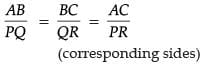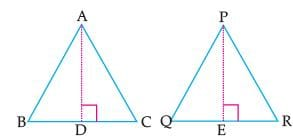∴ ∠B = ∠Q and ∠ADB = ∠PEQ (each 90°)

Now,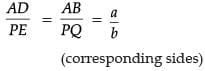## Mathematics (Maths) Class 10

115 videos|478 docs|129 tests
Information about Case Based Questions Test: Triangles - 2 Page
In this test you can find the Exam questions for Case Based Questions Test: Triangles - 2 solved & explained in the simplest way possible. Besides giving Questions and answers for Case Based Questions Test: Triangles - 2, EduRev gives you an ample number of Online tests for practice

## Mathematics (Maths) Class 10

115 videos|478 docs|129 tests